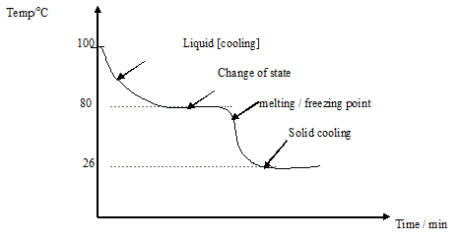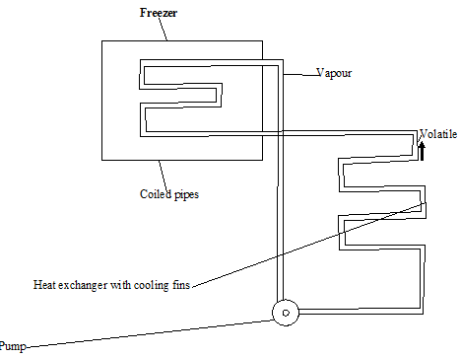# PHY4: LATENT HEAT

## LATENT HEAT

Latent heat is the energy released or absorbed, by a body or a thermodynamic system, during a constant-temperature process usually a first-order phase transition. Latent heat can be understood as energy in a hidden form that is supplied or extracted to change the state of a substance without changing its temperature.

### Latent heat and the kinetic theory

Change of state from solid to liquid is called melting or liquefaction while liquid to solid is called freezing or solidification.

During these changes of state, latent heat [hidden heat] is involved.

Kinetic theory suggests that when solid changes to a liquid, heat energy must be used to free molecule from the tactic force that holds them together hence heat is used or absorbed.During freezing heat energy is given out from the lattice bonds. A change from liquid to gases is called vaporization which from gas to liquid is condensation.

During vaporization heat is used to break the bond between molecules white during condensation heat energy is releases or given out.

• Find out why heat is given out

An experiment shows that when changes of state occurred the temperature of substances will always remain constant.

Latent heat of fusion

This is the quantity of heat required to convert a substance from solid to liquid without a change in temperatures.

Latent heat of vaporization

This is the quantity of heat required to convert a substance from liquid to gas state without a change in temperature.

These changes of state can be demonstrated by using the cooling process of liquid naphthalene.

This naphthalene is heated to 100oc in a water bath and then left to cool. The temperature is recorded every minute and a cooling curve of temperature against time is then drawn as follows;-NB:

The temperature remains constant as naphthalene solidifies.

Specific latent heat of fusion [Lf]

This is the quality of heat required to convert one kilogram of a substance from solid to the liquid state without change of temperature. S.1 unit is Joule per kilogram [Jkg-1].

The quantity [Q]of heat used on given out is the product of the mass and specific latent heat of fusion.

 Q = MLF

Specific latent heat of vaporization

This is the quantity of heat required to convert 1 kg of a substance from a liquid stat to a gases change without change in temperature.

 Q = MLF

Examples

An ice making machines removed from heat from water at a rate of 20 Js-1. How long will it take to convert 0.5 kg of water at 200c to ice at OOc

s.l.h of ice is 3.36 x 105 Jkg-1

Heat given out = MCO + mlf

= 0.5 x 4200 [20 -0] + 0.5 x 3.36 x 105

= 42,000 + 168,000

= 210,000 J

Rate of removal = 20 Js-1

Time             = Amount of Heat/ Rate

= 210,000/ 20

= 10,500 seconds

THIS VIDEO EXPLAINS THE SPECIFIC LATENT HEAT OF FUSION

The refrigeratorHow it works (Principles)

A refrigerator works on the principle that heat is taken into one point and given out at another point by the refrigerating substance as it is pumped around the circuit.

Cooling

The coiled pipes in the freezer contain a volatile liquid which takes latent heat from its surrounding causing it to cool.

Vapour

The latent heat taken from the surrounding enable the volatile liquid to change into a vapour.

Pump

The vapour formed is removed by the pump. This reduced the pressure resulting into the lowering of the boiling point of the volatile liquid.

Heat exchanger

The pump then forces the vapour into the heat exchanger where it is compressed and liquefies giving out the latent heat of vaporization.

Functions of the main parts of the refrigerator

Pump

1. The pump removes the vapour formed in the freezer
2. The pump forces the vapour into the heat exchanger

Heat exchanges

It’s where the vapour is compressed and liquefies giving out latent heat of vaporization

Cooling fin

The cooling fins give out latent heat of vaporization to the surrounding air.

Note

The cooling fins are pointed black so that they can quickly give out the latent heat during liquefying because black surface are good emitters of heat radiations.

THE VIDEO BELOW EXPLAINS MORE ABOUT LATENT HEAT

### ASSIGNMENT : LATENT HEAT assignmentMARKS : 10  DURATION : 1 week, 3 days

SEE ALL
•YOU